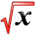# Simple Example of DerivativesThis article is part of the MathHelp Tutoring Wiki

## Implicit Differentiation (An example)

Q Differentiate $y=arccos(2t/(1+t^{2}))$A A trick to do this question is to convert the question to $cos(y)=2t/(1+t^{2})$. Now do implicit differentiation to get $-sin(y)y'=(-2t^{2}+2)/(1+t^{2})^{2}$. Since as know $cos(y)=2t/(1+t^{2})$, then $sin(y)=(t^{2}-1)/(t^{2}+1)$. (there're two ways to get this. One way is to use trig identity $sin^{2}+cos^{2}=1$; the other way is to draw a right-angled triangle with angle $y$, adjacent side $=2t$and hypotenuse $=(1+t^{2})$. Then the opposite side is $(t^{2}-1)$, and $sin(y)=opp/hyp$. So going back to the derivative and isolate $y'=(-2t^{2}+2)/(t^{4}-1)$.

Q Difference between secant and a tangent:

A A secant is any line passing through a graph. It would intersect the graph at least two points. However when two such points get closer and closer to each other, the secant becomes a tangent to a graph. One particular application is finding a derivative or slope of tangent at certain point. First find slope of the secant at $(x,y)$and $(x+h,y+h)$and then take limit $h->0$Q Find the derivative of $f(x)=x/(1+2x)$using the definition of limits

A $f'(x)=lim(h->0)1/h*(x+h/(1+2(x+h)-x/(1+2x))=lim(h->0)h/h*(1+2x)(1+2(x+h)=1/(1+2x)^{2}$Q find the tangent to the graph f(x) at x=a

A slope of tangent = f'(x), equation of tangent line is $y-f(x)=f'(x)(x-a)$[using the formula $y-y1=m(x-x1)$]

Q Find the derivative of $f(x)=2^{ex}e^{2x}$A Using the product rule we can write $f'(x)=2^{ex}(e^{2x})'+(2^{ex})'e^{2x}$.

Now the derivative of $e^{2x}$will be $2e^{2x}$using chain rule

Derivative of $2^{ex}=e\log(2)2^{ex}$using chain rule

So $f'(x)=2^{ex}2e^{2x}+e\log(2)2^{ex}e^{2x}$Hint to find derivative of $2^{ex}$: Derivative of $a^{x}$is $a^{x}\log a$using chain rule. use this formula $a^{x}=e^{x\log a}$. An extra $e$will come by derivative of $ex$COMMENT Revenue=Price*Number of Sales